• C语言怎么printf输出字符串。void main(){unsigned char *x = "ab,sdf,sad,23432,cc";data = x;printf("data = %c\n", *data);printf("data = %s\n", data);system("pause");}扩展资料：printf输出字符串的一些...
C语言怎么用printf输出字符串。void main(){unsigned char *x = "ab,sdf,sad,23432,cc";data = x;printf("data = %c\n", *data);printf("data = %s\n", data);system("pause");}扩展资料：printf输出字符串的一些格式1、原样输出字符串：printf("%s", str);2、输出指定长度的字符串, 超长时不截断, 不足时右对齐：printf("%Ns", str);注：N 为指定长度的10进制数值3、输出指定长度的字符串, 超长时不截断, 不足时左对齐：printf("%-Ns", str);注：N 为指定长度的10进制数值4、输出指定长度的字符串, 超长时截断, 不足时右对齐：printf("%N.Ms", str);注：N 为最终的字符串输出长度M 为从参数字符串中取出的子串长度5、输出指定长度的字符串, 超长时截断, 不足时左对齐是：?printf("%-N.Ms", str);注：N 为最终的字符串输出长度M 为从参数字符串中取出的子串长度参考资料来源：printf-百度百科关于C++习题，输入一串包涵标点符号的字符串变量，要求把中间的标点符号去掉后输出，写的代码的问题更改后的代码, 没有增删,只是把外边的cout>s) { cout<将一个字符串数组通过连接符号连接,并输出string tel="";//定义个字符串 for(i=0;i这段程序哪有问题，输出一段字符串里面以标点符号或者空格隔开的个数最长的回文，包括标点符号或空格一个小问题你的程序中：char s1,s2,s3;数组s1,s2,s3声明时没有初始化，导致最后输出结果时，将s3中没有被赋值的成员中的那些随机值也作为有效值输出了。这么改就可以了：char s1={NULL},s2={NULL},s3={NULL};改过以后的代码：#include#includevoid main(){char s1={NULL},s2={NULL},s3={NULL};int i,l,l2,l3,j,s=0;gets(s1);fflush(stdin);l=strlen(s1);for(i=0;i{l2=0;if(s1[i]!=' '&&s1[i]!=','&&s1[i]!='.'){s2[s++]=s1[i];l2++;}elsefor(j=0;j{if(s2[j]!=s2[l2-1-j]){s=0;break;}}l3=strlen(s3);if(j==(l2-1)/2&&(l2+1)>l3){s2[s++]=s1[i];s2[s]='\0';strcpy(s3,s2);s=0;continue;}else {i++;s=0;continue;}}printf("%s",s3);}
展开全文• 字符就是符号、图案，在计算机中以整数形式存储，当需要显示时会根据ASCII表中的对应关系，显示相应的符号或图案。 字符类型（char）的数据（如字符A，a，0，$等）在内存中以对应的ASCII码存放，不同字符所对应的... 字符 什么是字符 字符就是符号、图案，在计算机中以整数形式存储，当需要显示时会根据ASCII表中的对应关系，显示相应的符号或图案。 字符类型（char）的数据（如字符A，a，0，$等）在内存中以对应的ASCII码存放，不同字符所对应的ASCII码见下表。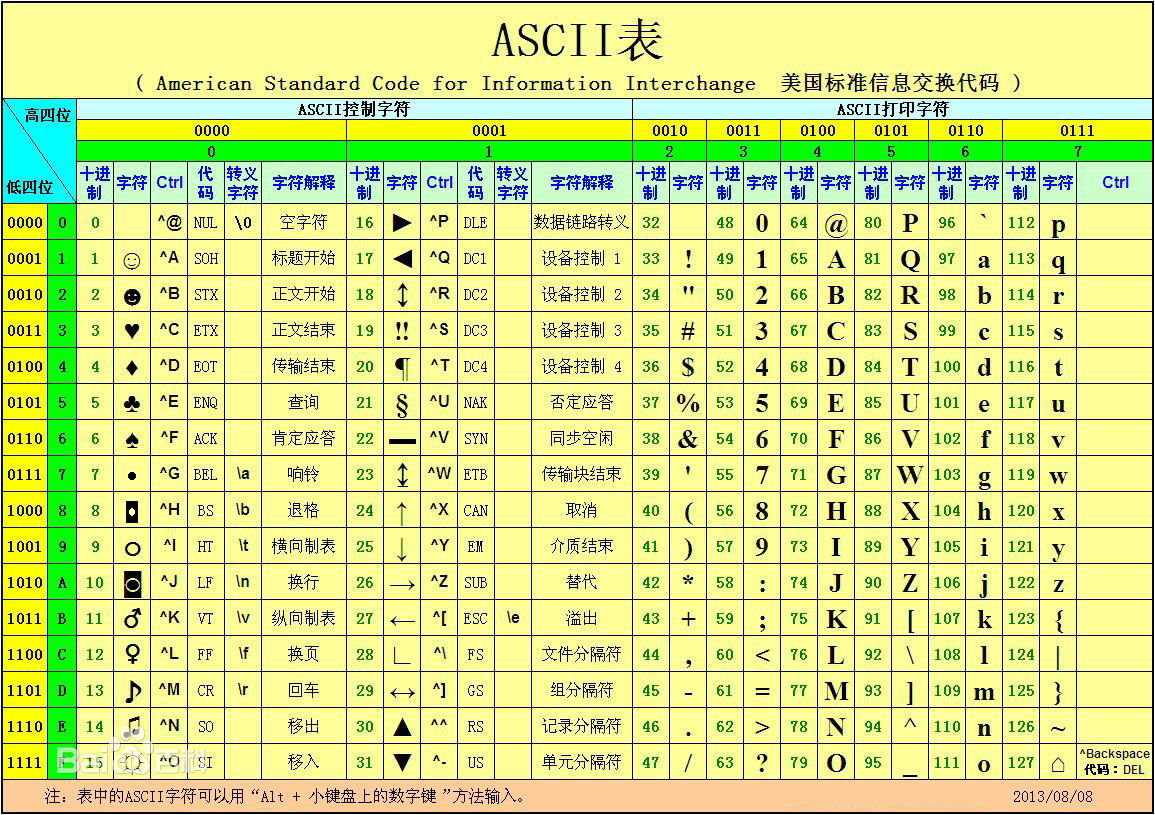计算机用一个字节（8个二进制位）存储一个字符，例如，字符A的ASCII码为65，它在内存中以如下形式存放：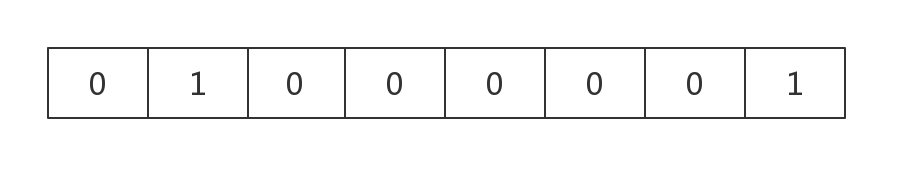单个字符的输入/输出函数
除了可以使用scanf()函数、printf()函数处理单个字符的输入/输出，在函数调用的格式控制字符串中对应的格式说明符用%c外，C语言还提供了只能处理单个字符的输入输出函数getchar()和putchar()。
字符输出函数：putchar()
#include<stdio.h>
int main()
{
char c = 'A';
putchar(c);
putchar('A');
putchar('\n');			//输出换行符
putchar('\101');		//八进制对应的字符A
putchar(65);			//十进制对应的字符A
}
/**
执行:
AA↙
AA
**/

注意：putchar()函数的参数可以是字符数据（包括转义字符）、整型数据，将该值看成某字符的ASCII码，输出对应字符。
字符输入函数：getchar()
#include<stdio.h>
int main()
{
char c1, c2, c3;
c1 = getchar();
c2 = getchar();
c3 = getchar();
putchar(c1);
putchar(c2);
putchar(c3);
}
/**
输入：
a b c↙
输出：
a b
**/

注意：空格符、制表符和回车符都是合法的ASCII字符，在输入时会被当做普通字符被接收。
字符串
什么是字符串
字符串是由字符组成的串型结构，它的结束标志是’\0’。其中的字符可以包含字符、数字、其他字符、转义字符、汉字。
字符串的定义和初始化
在C语言中，字符串存储在一维字符数组中。每个字符串常量有一个字符串结束标志’\0’隐藏在串最后，标志着该字符串结束。
char str = {'C','H','I','N','A','\0'};
//也可以用字符串初始化数组，上述初始化等价于
char str = {"CHINA"};
//或
char str = "CHINA";
//如果给出了全部元素的初值，则可以省略长度，长度会根据字符串自动调整
char str[] = "CHINA";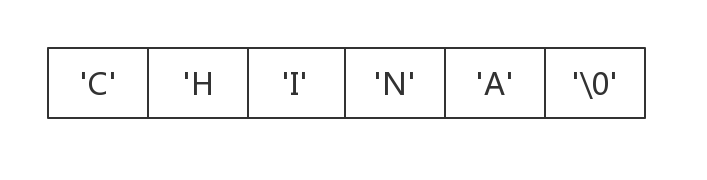未初始化或赋初值的数组元素值不确定：
char a;
a = 'C',a = 'H',a = 'I',a = 'N',a = 'A';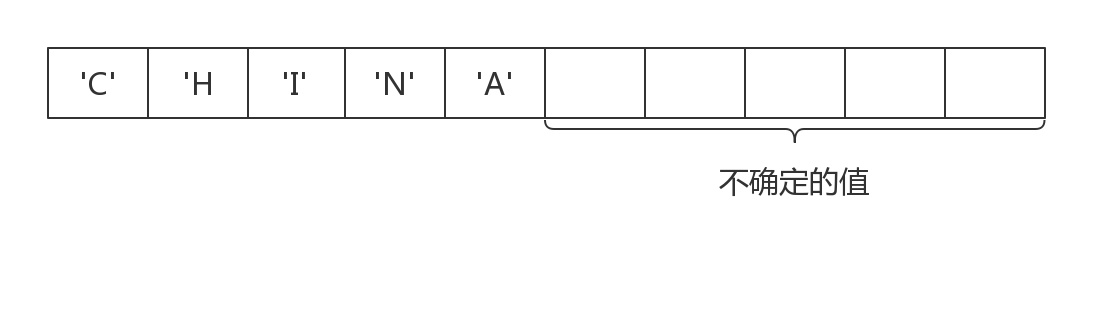初始化的数组，元素值默认为’\0’:
char a = "CHINA";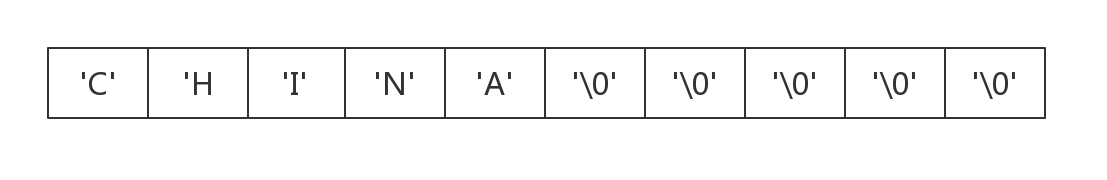注意：

数组的长度必须比字符串的元素个数多1，用来存储字符串的结束标志’\0’。

数组名是地址常量，不能将字符串直接赋值给数组名。
char c;c="CHINA" 非法的

字符串到第一个’\0’结束，所以常用’\0’判断字符串是否处理完毕。
char c = "abc\0def" 字符串实际存放的是"abc";

字符串存储在只读段，不能被修改。一般常用字符串字符值来初始化字符数组，编译器会自动把字符串字面值拷贝到数组中，包括’\0’，之后这个字符串就有了两份，一份在栈中，另一份在只读段。
int main()
{
char* str = "hehehehe";
str = 'H'; 		// error
}


字符串的输出/输入
字符串输出函数
printf()和puts()会逐个输出字符串中每个元素，知道遇到’\0’。
printf("%s",str);
puts(str) 		//会自动在末尾添加一个'\n'。

字符串输入函数
//scanf()用格式符"%s"输入若干个字符（可以是汉字）到字符数组，遇到空格、制表符或回车符终止，并写入'\0'。
scanf("%s",str);
//gets()读入一串以回车结束的字符（可以接收空格），顺序存入到以str为首地址的内存单元中，并在最后写入'\0'。
gets(str);
//fgets()能接受到size-1个字符（要为'\0'预留一个）。注意：当输入的字符不足size-1个时会连'\n'一起接收。
fgets(str,size,stdin);

字符串与指针
字符串一般存储在字符数组中，对字符串的访问可以逐个访问数组元素中的字符，或者利用指针访问字符串。

字符串指针指向字符串的首字符，它实际上是字符指针，其对象是字符。
字符串通常存储在字符数组中，其数组名就是这个字符串的指针。

常用字符串的函数
头文件：string.h

strlen
功能：计算字符串中字符的个数（不计’\0’）。
C语言源码：

	size_t __cdecl strlen (const char * str)
{
const char *eos = str;
while( *eos++ );
return( (int)(eos - str - 1) );
}


strcat
功能：把字符串2包括（’\0’），复制到字符串1后，函数返回字符串1。
C语言源码：

	char * __cdecl strcat (char * dst,const char * src)
{
char * cp = dst;
while( *cp )
cp++;
while( *cp++ = *src++ ) ;
return( dst );
}


strcpy
功能：字符串2复制到字符串1中，从字符串1指针指的位置开始依次存储字符串2，函数返回值为字符串1指针。
C语言源码：

	char * __cdecl strcpy(char * dst, const char * src)
{
char * cp = dst;
while( *cp++ = *src++ );
return( dst );
}


strcmp
功能：比较字符串1和字符串2两个字符串的大小。两字符串相同则函数返回值为0；字符串1>字符串2则返回值为1；否则返回-1。
C语言源码：

int __cdecl strcmp (const char * src,const char * dst)
{
int ret = 0 ;
while( ! (ret = *(unsigned char *)src - *(unsigned char *)dst) && *dst)
++src, ++dst;
if ( ret < 0 )
ret = -1 ;
else if ( ret > 0 )
ret = 1 ;
return( ret );
}


atoi, atol, atoll
功能：将字符串转为整数。逐个将字符串转为整数，如果有非整数字符，则终止。
C语言源码：

long __cdecl atol(const char *nptr)
{
int c;
long total;
int sign;
while ( isspace((int)(unsigned char)*nptr) )
++nptr;

c = (int)(unsigned char)*nptr++;
sign = c;
if (c == '-' || c == '+')
c = (int)(unsigned char)*nptr++;

total = 0;

while (isdigit(c)) {
total = 10 * total + (c - '0');
c = (int)(unsigned char)*nptr++;
}

if (sign == '-')
return -total;
else
return total;


strstr
功能：在str1中查找str2。如果有，返回str2在str1中首次出现的位置下标，如果没有返回NULL。
C语言源码：

char * __cdecl strstr (const char * str1,const char * str2)
{
char *cp = (char *) str1;
char *s1, *s2;
if ( !*str2 )
return((char *)str1);
while (*cp)
{
s1 = cp;
s2 = (char *) str2;
while ( *s1 && *s2 && !(*s1-*s2) )
s1++, s2++;
if (!*s2)
return(cp);
cp++;
}
return(NULL);
}



展开全文• 1： 什么字符串双引号括起来的0个或多个字符。"123" 2： 字符串的结束符号'\0' '\0'它是一个字符，不是一个字符串 3： 字符串输出： printf("%s",数组名); puts(字符串); 输入： scanf(); 或者 ...
一、指向字符串的指针

1： 什么是字符串？

用双引号括起来的0个或多个字符。"123"
2： 字符串的结束符号'\0'
'\0'它是一个字符，不是一个字符串
3： 字符串的输出：
printf("%s",数组名);
puts(字符串);

输入：

scanf();       或者 gets();
4:  strlen:用来测量一个字符串中所包含的字符个数

二、 指向变量的指针：

int a=3,*p=&a;  p--->a
指向一维数组元素的指针：
int a={1,2,3,4},*p=a;  p--->a=1
指向一维数组的指针：
int a,(*p)=a;   p--->a---->a
一个指针也可以指向字符串：
char *指针名;
char *p;  定义了一个指针p以后这个指针可以指向一个字符串
如何给指向字符串的指针赋初值：
1： 在定义的时候赋初值
char *p="abcd";
2： 在定义之后赋初值
char *p;
p="abcd";
p---->a
b
c
d
\0
说明：
1）  可以把字符串直接赋给一个指针
2）  但是这并不是说吧字符串中的所有字符都赋给了p，而事实上指针p永远只能指向一个字符
指针p一开始指向字符串的第一个字符
3） 一般经常把一维字符型数组与指向字符串的指针结合
4 ) 数组名a是常量，不能自加，而指针p可以移动，p是变量
5 ) 如何把一个指向字符串的指针移动到一个数组的末尾           p=a+strlen(a)-1;
6）讨论 *p
7） 指向字符串的指针就是一个字符串
char *p="abcd"        我们可以说p就是"abcd"
8)  如何通过指针来访问每一个字符：
p=数组名；

while(*p!= '\0')或者while(*p)
{
p++;
}
比如：讨论 *p
char a="1234",*p=a;   //定义时候的* 是一个指针标识，以后使用的时候p是指针，  *p就是a,是一个字符
printf("%c",*p);//*p是一个字符
printf("%s",*p);//错误
printf("%s",p); //p指向字符串
a---->1<----p
2<----p+1
3
4
\0
4) 数组名a是常量，不能自加，而指针p可以移动，p是变量
指向字符串的指针的输出：
puts(指针名);
printf("%s",指针名)；
%c输出的是字符
%s输出的是字符串
5) 以字符串作为函数参数的几种格式：
A:型参与实参都用数组
型参： fun(char a[])
实参： fun(a)

B:型参用数组，实参用指针
型参： fun(char a[])
实参： fun(p)//p是指针

C:型参用指针，实参用数组
型参： fun(char *p)   p可以当成数组使用
实参： fun(a)//a是数组名

6) 一维字符型数组就是字符串
7) 以数组作为函数的参数，型参数组就是实参数组，实参数组就是型参数组，因为数组名是首地址，实参把数组的地址传给了实参数组，传址关系  。
输出的原理：
以当前指针的位置开始，一直向下输出，直到遇到 \0 为止

 main()
{
char a={"abcd123"},*p;
p++;
puts(p);
puts(a);
printf("%s",p);
printf("%d",strlen(p));
p=a+strlen(a)-1;    //就可以让指针p直接指向数组的最后一个元素

}

char a={"abcd123"},*p=a;

while(*p!= '\0')
{
p++;
}


指向字符串指针应用举例
strlen(s): 用来返回字符串含有的字符个数，不包含'\0'
sizeof(a): 用来返回一个数组的大小,如果是动态数组，包含最后的\0 指向同一个数组元素的两个指针可以相减，相减的结果是两个指针中间所含有的元素个数。

include "string.h"
void main()
{
char s={"\n123\\"};//s[]
printf("%d\n",strlen(s));//5
printf("%d",sizeof(s)); //100,c 程序会为s分配100个单位的内存地址
}

void main()
{
char s[]={"\n123\\"};    //s[]动态数组，会根据实际情况来分配内存单元
printf("%d\n",strlen(s));//5
printf("%d",sizeof(s));  //6
}

void main()                                  str--->A<---p
{                                                   B
char str[]="ABCD,*p=str;                    C
printf("%d\n",*(p+4));//0                   D
printf("%c\n",*(p+4));//                  \0<---p+4
}

void main()
{
char s[]="ABCD",*p;
for(p=s;p<s+4;p++)
printf("%s\n",p));

}
key		A
B
C
D
void main()
{
char str[]="xyz",*ps=str;
while(*ps)
{
ps++;
}
printf("%d",ps-str);
for(ps--;ps-str >= 0;ps--)
puts(ps);  //zyx

}
3
z
yz
xyz

void main()
{
char str[]={"hello","beijing"},*p=str;

printf("%d",strlen(p+20));//7
}
str -->  str--->h <---p
e
l
l
o
\0<---p到这里就跑不动了，下不去了。从这里往下数，后面正好是7个字符
str +1-->str-->b
e
i
j
i
n
j
\0

void main()
{
char s[]="159123",*p;
p=s;
printf("%c",*p++);//1
printf("%c",*p++);//5
printf("%s",p);//"9123"
}

%c  输出的是字符
%s  输出的是字符串

指向字符串的指针二

void fun(char *s,int p,int k)
{
int i;
for(i=p;i<k-1;i++)
{
s[i]=s[i+2];
}
}
main()
{
char s[]="abcdefg";
fun(s,3,strlen(s));
puts(s);//abcfg
}

-------------------------------------------------

void fun(char a[])//把字符串的每一个元素都加一
{
int i;
for(i=0;a[i];i++)
{
a[i]=a[i]+1;
}
}

void move(char *str,int n)//把字符串向右移动一位，并把末尾的字符放到首位。
{
int i;
char temp=str[n-1];//n=5,n表示从哪里开始移
for(i=n-1;i>0;i--)
{
str[i]=str[i-1];
}
str=temp;
}

main()
{
char s="abcde";
int n=3,i,z;
z=strlen(s);//5
for(i=1;i<=n;i++)//移动了3次
{
move(s,z);
}
printf("%s",s);//cdeab
}


一维字符型数组就是字符串

char *p;  这个指针也指向字符串
如果把一个数组的首地址赋给了某个指针，这个指针就可以当数组来使用。
fun(char *p)
{
p
}
main()
{
char a[]={"abcd"};
fun(a);
}
编写一个程序，统计一个字符串中所有字母的出现，不区分大小写
"abcdeabcder"
void fun(char *a,int *b)
{
while(*a)
{
b[*a-'a']++;
*a++;
}
}
void main()
{
}编写一个函数，它的功能是把字符串str下标为偶数位置上的字符，紧随其后重复出现一次，放在一个新串t中，且t中的字符要按顺序来存放
str="123456"  "113355"
t="553311"

void fun(char *str,char *t)
{
int i,j=0;
for(i=strlen(str)-1;i>=0;i--)//倒过来访问字符串的所有字符
{
if(i%2==0)
{
t[j++]=str[i];
t[j++]=str[i];
}
}
t[j]='\0';
}
main()
{
char st,t;
printf("请输入一个字符串：");
gets(str);
fun(str,t);
puts(t);
}

编写一个函数，目的是把一个字符串的所有单词第一个字母都变成大写字母
字符串的字符都是空格隔开的，并且第一个字符不是空格

"i am a student to take the test" ------>"I Am A Student To Take The Test"

//方法一

void fun(char *a)
{
int i=1,j=0;
if(a>='a' && a<='z')  //首字母单独处理
{
a-=32;
}
while(a[i])
{
if((a[i]>='a' && a[i]<='z') && a[i-1]==' ')

{
a-=32;
}
i++;
}
}

//方法二，用指针
void fun(char *a)
{
int i=1,j=0;
if(*a>='a' && *a<='z')
{
*a-=32;
}
while(*a)
{
if((*a>='a' && *a<='z') && *(a-1)==' ')

{
*a-=32;
}
a++;
}
}



题目：

编写一个函数，它的功能是将未在字符串s中出现，而在字符串t中出现的字符，型参一个新的字符串放在u中，
u中的字符按原字符的顺序排列，但要去掉重复字符
至少会想到去考察t，以t为标准
s="12345";
t="224677";
u="67"

//方法一：
void funa(char *s,char *t,char *u)
{
int i,j,k=0,m;
for(i=0;i<strlen(t);i++)
{
for(j=0;j<strlen(s);j++)
{
if(t[i]==s[j])//t[i]包含在s中
{
break;
}

}
if(j==strlen(s))//如果t[i]不存在于s中
{

if(k==0)//第一个时
{
u[k++]=t[i];
}
for(m=0;m<k;m++)//第二个开始检查u中是否已经存在
{
if(t[i]==u[m])
break;
}
if(m==k)
{
u[k++]=t[i];
}
}
}
u[k]='\0';

}

方法二：
int find(char *str,char findcahr)
{
int i;
for(i=0;str[i];i++)
{
if(str[i]==findchar)
return 1;
}
return 0;
}
void funa(char *s,char *t,char *u)
{
int i,j=0,k=0,m;
for(i=0;t[i];i++)//如果t[i]不在s中并且不在u中就放入到u中
{
if(findchar(s,t[i])==0 && findchar(u,t[i])==0)
{
u[j++]=t[i];//表示吧t[i]放入u[j]里面去
}
}
//退出循环后，应该把t[j]做成\0
u[j]='\0';

}
main()
{
char s="13745";
char t="2246778899";
char u="";
funa(s,t,u);
puts(u);
}


展开全文• 文章目录1、把整数/长整数格式化输出字符串2、注意事项3、版权声明 各位可能在网上看到以下函数可以将整数转换为字符串： itoa(); //将整型值转换为字符串 ultoa(); // 将无符号长整型值转换为字符串 请注意...
文章目录1、把整数/长整数格式化输出到字符串2、注意事项3、版权声明
各位可能在网上看到用以下函数可以将整数转换为字符串：
  itoa(); //将整型值转换为字符串
ultoa(); // 将无符号长整型值转换为字符串

请注意，上述函数与ANSI标准是不兼容的，很多编译器根本不提供这几个函数，本文就不介绍了，没什么意义。
将整数转换为字符串而且能与ANSI标准兼容的方法是使用sprintf()和snprintf()函数，在实际开发中，我们也是这么做的。
1、把整数/长整数格式化输出到字符串
标准的C语言提供了atoi和atol函数把字符串转换为整数和长整数，但是没有提供把整数/长整数转换为字符串的库函数，而是采用sprintf和snprintf函数格式化输出到字符串。
函数声明：
int sprintf(char *str, const char *format, ...);
int snprintf(char *str, size_t size, const char *format, ...);

示例（book98.c）
/*
* 程序名：book98.c，此程序演示格式化输出sprintf和snprintf函数。
* 作者：C语言技术网(www.freecplus.net) 日期：20190525
*/
#include <stdio.h>
#include <string.h>

int main()
{
int ii=1024;
long ll=12345678901234;
char strii,strll;

memset(strii,0,sizeof(strii)); memset(strll,0,sizeof(strll));

// 把整数ii转换为字符串，存放在strii中。
sprintf(strii,"%d",ii);
printf("strii=%s\n",strii);  // 输出strii=1024

// 把长整数ll转换为字符串，存放在strll中。
sprintf(strll,"%ld",ll);
printf("strll=%s\n",strll);  // 输出strll=12345678901234

memset(strii,0,sizeof(strii)); memset(strll,0,sizeof(strll));

// 把整数ii转换为字符串，存放在strii中，只保留前10个字符。
snprintf(strii,11,"%d",ii);
printf("strii=%s\n",strii);  // 输出strii=1024

// 把长整数ll转换为字符串，存放在strll中，只保留前10个字符。
snprintf(strll,11,"%ld",ll);
printf("strll=%s\n",strll);  // 输出strll=1234567890
}

运行结果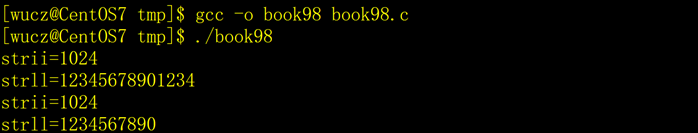2、注意事项
snprintf函数在unix和windows平台下的表现略有不同，在Linux平台下，保留size-1个字符，在windows平台下，保留size个字符。
3、版权声明
C语言技术网原创文章，转载请说明文章的来源、作者和原文的链接。
来源：C语言技术网（www.freecplus.net）
作者：码农有道
如果这篇文章对您有帮助，请点赞支持，或在您的博客中转发我的文章，谢谢。


展开全文• scanf函数的一般形式为：scanf("格式控制字符串",地址列表); scanf输入的是地址，printf输出的是值,如下举例说明 #include<stdio.h> void main() { int a,b,c; printf("input the value of a,b,c\n"); ...
• 输出学习时的笔记（其实也没什么用，留着给自己看的...1、格式占位符（格式占位字符串）%d 接收int型 输出有符号的10进制%f 接收float型 输出的时候 %f或者%lf是一样的%c 接收char型 输出字符%lf 接收double型 %s ...
• 变量的输出 变量如何输入输出呢？实际上，在这之前你已经使用...格式控制字符串用特定的格式说明符代表输出的数据类型，这里的数据可以是变量、常量或者结果值具有合适类型的表达式。 之前看到的符号%d有什么作用...
• 10.1. 用什么方法存储标志(flag)效率最高? 147 10.2. 什么是“位屏蔽(bit masking)”? 148 10.3. 位域(bit fields)是可移植的吗? 150 10.4. 移位和乘以2这两种方式中哪一种更好? 151 10.5. 什么是高位字节和低位字节...
• 字符类型 char 1~2000字节 固定长度字符串，长度不够的空格补充 varchar2 1~4000字节 可变长度字符串，与CHAR类型相比，使用VARCHAR2可以节省磁盘空间，但查询效率没有char类型高 数值类型 Number(m,n) m(1~38) ...
• 　本例中用一个循环语句给a数组各元素送入奇数值，然后第二个循环语句从大到小输出各个奇数。在第一个 for语句中，表达式3省略了。在下标变量中使用了表达式i++，以修改循环变量。当然第二个for语句也可以这样作...
• ## 你必须知道的495个C语言问题

千次下载 热门讨论 2015-05-08 11:09:25
1.3　因为C语言没有精确定义类型的大小，所以我一般都typedef定义int16和int32。然后根据实际的机器环境把它们定义为int、short、long等类型。这样看来，所有的问题都解决了，是吗？ 1.4　新的64位机上的64位类型...
• 15.5.3 scanf读入字符串 419 15.5.4 格式化i/o的一个实例 420 15.5.5 使用scanf的局限 423 小结 423 复习题 424 程序设计练习 425 第16章 记录 431 16.1 数据记录的概念 432 16.2 记录在c语言中的使用 432...
• 数组实现的字符串指针实现的字符串的不同点 318 字符串数组 320 11-2 通过指针操作字符串 323 判断字符串长度 323 字符串的复制 325 不正确的字符串复制 328 返回指针的函数 329 11-3 字符串处理...
• previous up contents next C 语言常见问题集 原著：Steve Summit 翻译：朱群英, 孙 云 修订版 0.9.4, 2005年6月23日... o 8.6 我有个函数, 本该返回一个字符串, 但当它返回调用者的时候, 返回串却是垃圾信息。 ...
• 2.4.4字符串常量 2.4.5宏常量 2.4.6枚举常量 2.5变量 2.5.1变量的定义与初始化 2.5.2const类型修饰符 2.5.3使用变量时的注意事项 2.6常用运算符及表达式 2.6.1运算符的优先级与结合性 2.6.2算术运算符 ...
• 6.1 什么字符串 201 6.2 处理字符串和文本的方法 203 6.3 字符串操作 206 6.3.1 连接字符串 206 6.3.2 字符串数组 208 6.4 字符串库函数 210 6.4.1 使用库函数复制字符串 210 6.4.2 使用库函数确定字符串...
• 书中列出了C用户经常问的400多个经典问题，涵盖了初始化、数组、指针、字符串、内存分配、库函数、C预处理器等各个方面的主题，并分别给出了解答，而且结合代码示例阐明要点。 《你必须知道的495个C语言问题》结构...
• 1.3　因为C语言没有精确定义类型的大小，所以我一般都typedef定义int16和int32。然后根据实际的机器环境把它们定义为int、short、long等类型。这样看来，所有的问题都解决了，是吗？　2　 1.4　新的64位机上的64位...C 进阶
• 输入学习时的笔记（其实也没什么用，留着给自己看的） 输出是指 把内容打印到控制台窗口 输入是指 把内容输入到程序里 scanfscanf 从键盘获取内容到程序里格式占位符+变量要加取地址符 1、格式占位符 %d 整数类型...
• 作者在网络版CFAQ列表的基础上进行了大幅度的扩充和丰富，结合代码示例，权威而且详细深入地解答了实际学习和工作中最常遇到的495个C语言问题，涵盖了初始化、数组、指针、字符串、内存分配、库函数、C预处理器等...C C++
• 1.3　因为C语言没有精确定义类型的大小，所以我一般都typedef定义int16和int32。然后根据实际的机器环境把它们定义为int、short、long等类型。这样看来，所有的问题都解决了，是吗？ 1.4　新的64位机上的64位类型...# c语言输出字符串用什么符号c语言 订阅